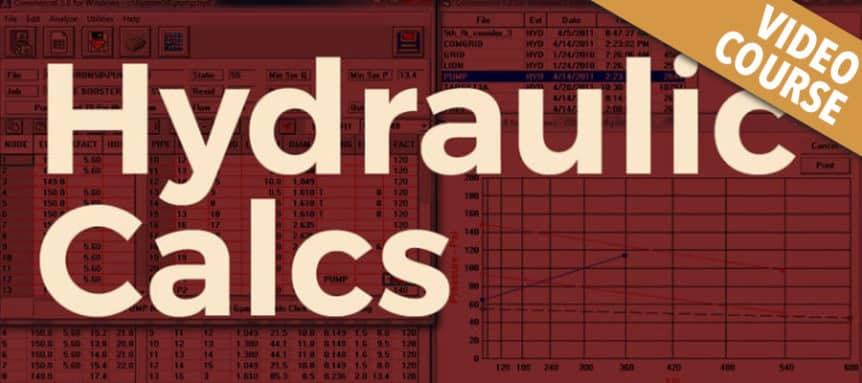Hydraulic Calculations (CA)

Tanya Haun

Current Status
Not Enrolled
Price
\$\$105
Get Started

Course Description:

This interactive seminar covers the requirements for properly hydraulically calculating a water-based fire protection system in accordance with NFPA-13. The seminar clearly addresses the basic hydraulic formulas utilized, their appropriate application, and various modifications to the calcs required or allowed by the NFPA-13 standards. We will cover and address the various hydraulics tables used in the standard, their specific purposes, and when to use which tables based on system type and application. The attendees will be involved in a significant number of exercises addressing various problems encountered in hydraulic calculation, how to identify when there is a problem with the hydraulics and learn various methods on correcting the identified problems in the calcs. Learning outcomes: At the conclusion of this seminar the participant will be able to:

1. Explain the definitions and equations relative to the hydraulic calculation process.
2. Explain the basis hydraulic principles at play.
3. Explain and perform the basic hydraulic calculation process.
4. Review hydraulic calculations for accuracy and completeness.
5. Understand the basic hydraulic laws of hydrostatics & hydrokinetics.
6. Discuss the various ways flow and pressure act upon the piping and pipe size.
7. Discuss and explain static and residual pressures and the difference between them.
8. Apply the various tables in the standard to the appropriate situation.
9. Explain the effect of elevation pressure to the hydraulic calculations process.
10. Properly calculate velocity pressures created by water moving thru pipes.
11. Understand how the flow of water affects/is affected by the velocity (speed) of the flow.
12. Calculate a small sprinkler branch-line by hand.
13. Understand the primary path of water in a set of hydraulic calculations.
14. Properly review a complete set of hydraulic calculations.

3 Hours of Continuing Education (.3 CEUs)
Instructor:
Ray Vance

This course is approved for:

• California Sprinkler Fitter

This course requires that a minimum total time of 180 minutes is spent in the coursework. This course has been divided into 5 separate video sessions to deliver the course content. When you are in a lesson, you must complete the video content before you will be permitted to proceed to the next page. If you need to take a break, you can do so in between each lesson.

The lessons are set to a minimum time but you can take as much time as you need beyond that. Don’t rush through the material in the lessons and feel free to take notes.

Click on the first lesson below to proceed to the first video.